# For the state of plane stress shown, determine the largest value of σY for which the maximum in-plane shearing stress is equal to or less than 75 MPa.

Question-AnswerCategory: Strength of MaterialsFor the state of plane stress shown, determine the largest value of σY for which the maximum in-plane shearing stress is equal to or less than 75 MPa.

For the state of plane stress shown, determine the largest value of σY for which the maximum in-plane shearing stress is equal to or less than 75 MPa.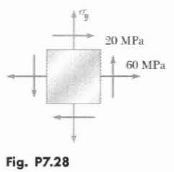Step No: 1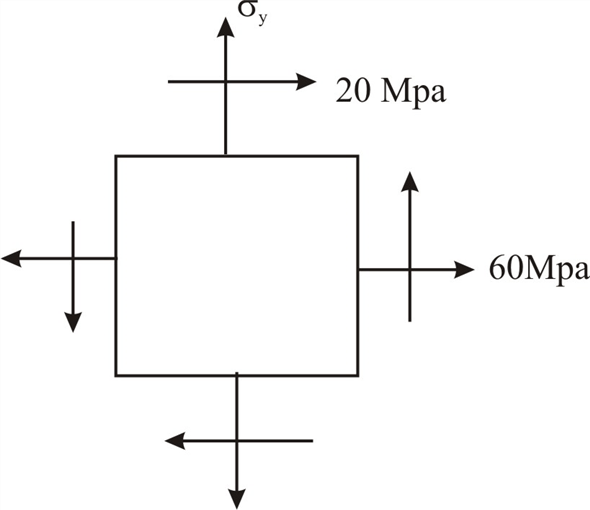Step No: 2

Tensile stress in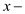direction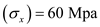Shear stress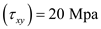Shear maximumStep No: 3

Let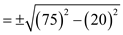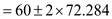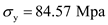Largest value is consider so